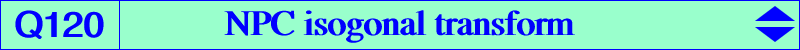∑ a^4 SA y^2 z^2 = a^2 b^2 c^2 x y z (x + y + z)X(59), X(249), X(250), X(2065), X(10419) extraversions of X(59) infinite points of MacBeath circumconic other points belowQ120 is the isogonal transform of the nine point circle (N) and the GSC transform of the circumcircle. In other words, for any M on the circumcircle, GSC(M) = X(3)-cross conjugate of M lies on Q120. Q120 is a circular quartic with singular focus X(2070), with three nodes at A, B, C where the nodal tangents concur at O and K. The fourth point Oa of Q120 on the A-cevian line of O is the isogonal transform of the midpoint of AH, obviously on (N). The fourth point Ka of Q120 on the A-symmedian is the isogonal transform of the second point of (N) on the median AG. These six points Oa, Ob, Oc, Ka, Kb, Kc lie on a same conic (C). Locus property : A variable line L passing through O meets the sidelines of ABC at A', B', C'. Let A", B", C" be their respective inverses in (O). The lines AA", BB", CC" concur at Q on Q120. It follows that the circles OAA', OBB', OCC' are in a same pencil. They meet at O and again at the inverse P of Q in (O). The locus of P is a tricircular sextic (see ADGEOM #3688).